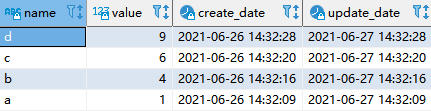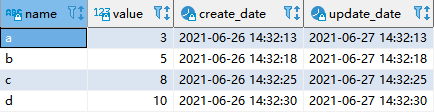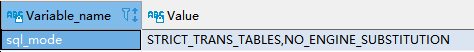*爱可生开源社区出品，原创内容未经授权不得随意使用，转载请联系小编并注明来源。

``````select name, value, create_date, update_date from t1 group by name order by create_date desc;
````````````select * from (select name, value, create_date, update_date from t1 order by create_date desc) t group by t.name;
````````````select name, value, create_date, update_date from t1 group by name order by create_date desc;
````````````select * from t1 a where create_date = (select max(create_date) from t1 b where a.name = b.name);
``````

``````select * from t1 a where not exists (select * from t1 b where a.name = b.name and b.create_date > a.create_date);
``````

MySQL 8.0支持 row_number()函数，操作应该和如下 Oracle 相近的。

Oracle 中可以使用 row_number()实现此需求：

``````select * from (select name, create_date, row_number() over (partition by name order by create_date desc) as r from t1) where r=1;
``````100
Subscribe